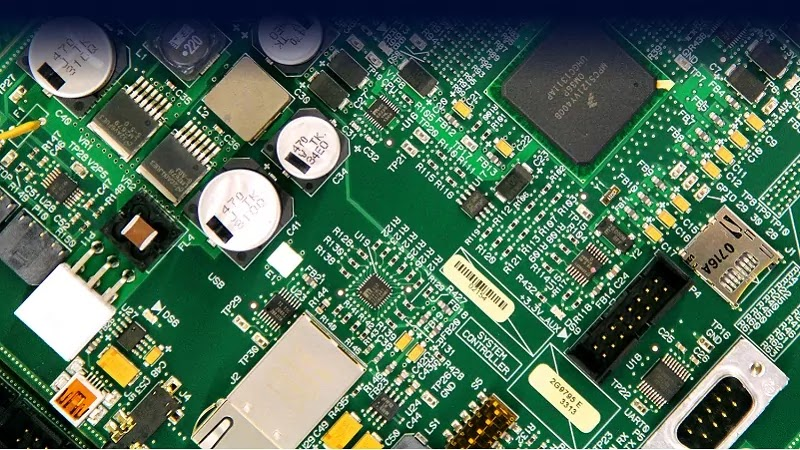Fig 1:  OP-AMP: Everything you need to know as an Engineer

In the following article, we'll be covering all the topics of Operational Amplifier. The topics covered here are the introduction to an op-amp, need for an op-amp, open-loop gain, voltage transfer curve of op-amp and equivalent circuit of an op-amp.

### INTRODUCTION OF AN OP-AMP

The operational amplifier is basically an amplifier that is abbreviated as an opamp. An amplifier's basic use is to amplify a signal. The same goes with this but here is a twist...

Why do we need an Op-Amp?

In the early days, when digital computers have not developed the operation like addition, subtraction, multiplication, division, integration and differentiation were performed using an op-amp by connecting a few resistors and capacitors it is ready to do this job.Fig 2: Symbol of an Op-Amp

According to the diagram if cane off of this operational amplifier is considered as 'A'. Then the output will be " A (V1 - V2) ". Hence this amplifier amplifies the difference between the two inputs.

#### Open Loop Gain

Open-loop gain is the gain of an operational amplifier when there is no feedback from the output to the input side. This condition occurs when one of the two input is grounded.

#### Voltage Transfer Curve of an Op-ampHere, the output voltages of an opamp are restricted or somehow controlled by the biasing voltages that are being applied to the op-amp so the output voltage will be between the biasing voltages i.e. +Vsat and -Vsat.

So whenever an opamp is used in an open-loop configuration that means there is no feedback from the output to the input side, at that time even if we apply a small input between these terminals, then also you will find that the output gets saturated towards the positive or negative biasing voltages.

And this particular characteristic is useful when we use it as a comparator.

#### Applications of an Op-amp

1. Anupam is useful in designing active filters.

2. Can be used in the construction of oscillators.

3. Waveform converters.

4. Analogue digital converters.

#### Equivalent circuit of an Op-ampFig 4: Equivalent Circuit diagram of an Op-amp

Here,

Ri is the input impedance of this op-amp

Ro is the output impedance of this op-amp

And output voltage will be the open looking multiplied with the difference between input V1 and V2.

So this up for today guys... the rest we will cover in our upcoming articles. Till then keep learning from

OYETECHY.COM

For Covid 19 updates and vaccine registration you can reach out to

COVID-19 UPDATES || Covid Relief || India Covid Support || Verified Leads

Note: For any queries or questions you can directly mail us. Or just dm me on Instagram @chakit_gupta.

OP-AMP: Everything you need to know as an EngineerReviewed by Oyetechy.com on May 23, 2021 Rating: 5